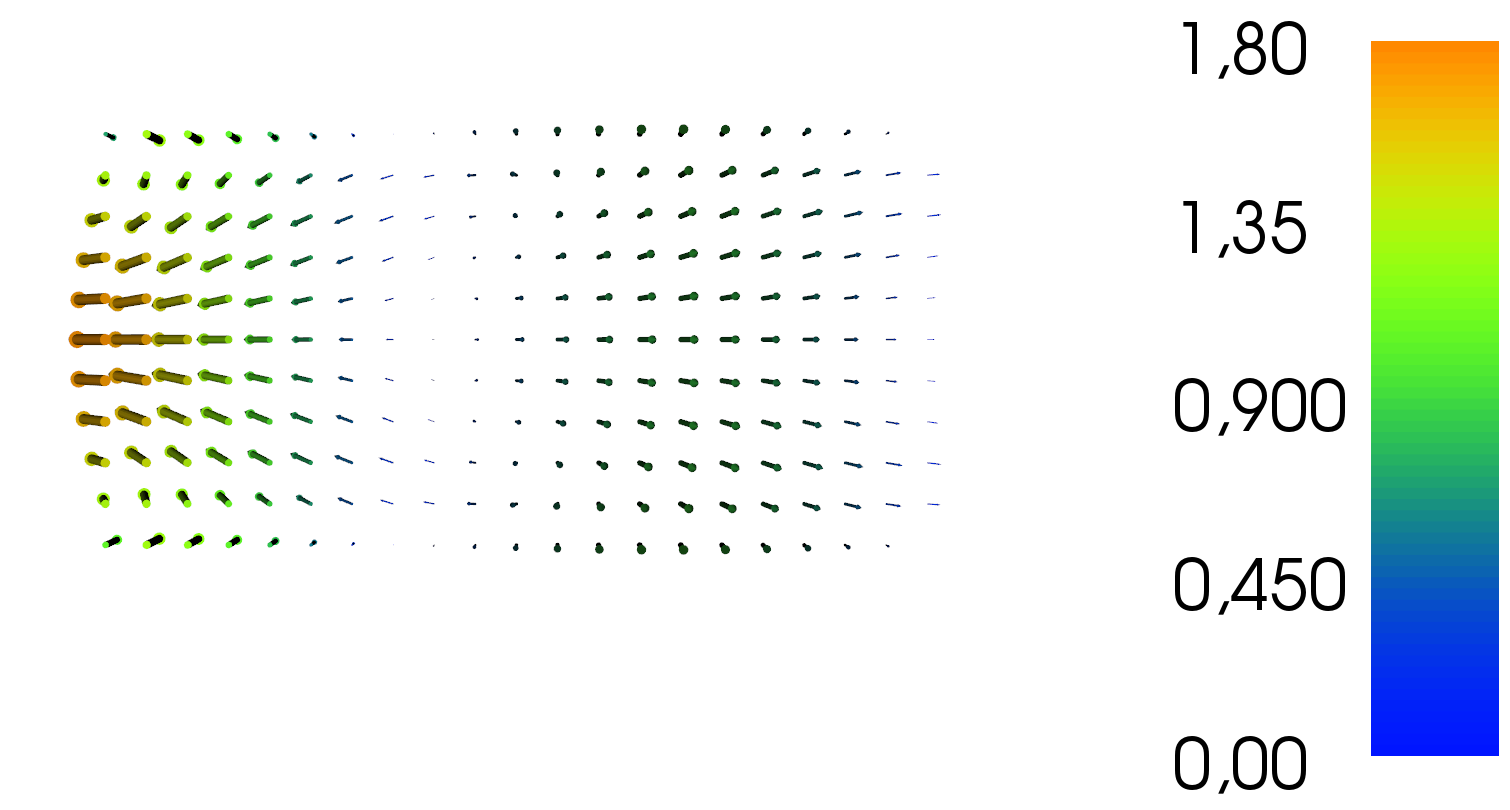# 2.1. Sinusoidal wave in a channel¶

## 2.1.1. Introduction¶

This example simulates the flow in a channel with oscillating velocity in-/outflow on the west boundary, fixed surface height on the east boundary, and free-slip flow on the north and south boundaries.

It shows how to:
• create a rectangular domain;
• specify velocity and surface elevation boundary conditions;
• set up a and solve the shallow water solver;
• plot the results.

The shallow water equations to be solved are

$\begin{split}\frac{\partial u}{\partial t} + u \cdot \nabla u - \nu \nabla^2 u + g \nabla \eta + \frac{c_b}{H} \| u \| u = 0, \\ \frac{\partial \eta}{\partial t} + \nabla \cdot \left(H u \right) = 0, \\\end{split}$

where

• $$u$$ is the velocity,
• $$\eta$$ is the free-surface displacement,
• $$H=\eta + h$$ is the total water depth where $$h$$ is the water depth at rest,
• $$c_b$$ is the (quadratic) natural bottom friction coefficient,
• $$\nu$$ is the viscosity coefficient,
• $$g$$ is the gravitational constant.

The boundary conditions are:

$\begin{split}u = \begin{pmatrix}\sin(\frac{\pi t}{5}) \frac{y (50-y)}{625}\\0\end{pmatrix} & \quad \textrm{on } \Gamma_1, \\ \eta = 0 & \quad \textrm{on } \Gamma_2, \\ u \cdot n = 0 & \quad \textrm{on } \Gamma_3, \\\end{split}$

where $$n$$ is the normal vector pointing outside the domain, $$\Gamma_1$$ is the west boundary of the channel, $$\Gamma_2$$ is the east boundary of the channel, and $$\Gamma_3$$ is the north and south boundaries of the channel.

After a few timesteps the solution should looks like this:## 2.1.2. Implementation¶

We begin with importing the OpenTidalFarm module.

from opentidalfarm import *
set_log_level(ERROR)  # Tell solver to only print error messages


Next we get the default parameters of a shallow water problem and configure it to our needs.

prob_params = SWProblem.default_parameters()


First we define the computational domain. For a simple channel, we can use the RectangularDomain class:

domain = RectangularDomain(x0=0, y0=0, x1=100, y1=50, nx=20, ny=10)


The boundary of the domain is marked with integers in order to specify different boundary conditions on different parts of the domain. We create a plot of these facets ids to inspect the boundary ids with (open the file facet_ids.xdmf in [Paraview](http://www.paraview.org/) to view the plot):

XDMFFile("facet_ids.xdmf").write(domain.facet_ids)


Once the domain is created we attach it to the problem parameters:

prob_params.domain = domain


Next we specify boundary conditions. For time-dependent boundary condition use a parameter named t in the dolfin.Expression and it will be automatically be updated to the current timelevel during the solve.

bcs = BoundaryConditionSet()
u_expr = Expression(("sin(pi*t/5)*x*(50-x)/625", "0"), t=Constant(0))

# Apply a strong no-slip boundary condition. This can be changed to
# free slip (weakly enforced), by leaving out the Constant((0, 0))
# argument and changing bctype to "free_slip"


Again we attach boundary conditions to the problem parameters:

prob_params.bcs = bcs


The other parameters are straight forward:

# Equation settings
prob_params.viscosity = Constant(30)
prob_params.depth = Constant(20)
prob_params.friction = Constant(0.0)
# Temporal settings
prob_params.theta = Constant(0.5)
prob_params.start_time = Constant(0)
prob_params.finish_time = Constant(25)
prob_params.dt = Constant(0.5)
# The initial condition consists of three components: u_x, u_y and eta
# Note that we do not set all components to zero, as some components of the
# Jacobian of the quadratic friction term is non-differentiable.
prob_params.initial_condition = Constant((DOLFIN_EPS, 0, 0))


Here we only set the necessary options. A full option list with its current values can be viewed with:

print prob_params


Once the parameter have been set, we create the shallow water problem:

problem = SWProblem(prob_params)


Next we create a shallow water solver. Here we choose to solve the shallow water equations in its fully coupled form. Again, we first ask for the default parameters, adjust them to our needs and then create the solver object.

sol_params = CoupledSWSolver.default_parameters()
sol_params.dump_period = -1
solver = CoupledSWSolver(problem, sol_params)


We create some files to store the velocity, u, and surface elevation, eta, at each timestep, so it can be viewed in ParaView later.

f_u = XDMFFile("u.xdmf")
f_eta = XDMFFile("eta.xdmf")


Now we are ready to solve the problem.

for s in solver.solve():
print "Computed solution at time {}".format(s["time"])

# Write velocity and free-surface perturbation to file.
f_u.write(s["u"], s["time"])
f_eta.write(s["eta"], s["time"])


The inner part of the loop is executed for each timestep. The variable s is a dictionary and contains information like the current timelevel, the velocity and free-surface functions.

## 2.1.3. How to run the example¶

The example code can be found in examples/channel-simulation/ in the OpenTidalFarm source tree, and executed as follows:

\$ python channel-simulation.py


The results are stored in the files u.xdmf and eta.xdmf, and can be viewed with [Paraview](http://www.paraview.org/).